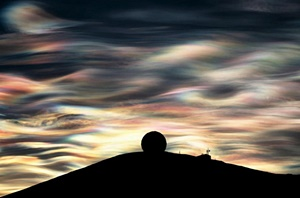Low Level Artificial "Ionosphere" Propagation Model
Objective : Propagate AC Electromagnetic Field to Remote Location

This model will estimate:
1.Electron Density per Cubic Centimeter (low level-"ionosphere")
2. Skip Distance of Reflective Layer in Miles (low level- "ionosphere").
3. Attenuated Power in mW/m^2 at Skip Distance using Free Space propagation.
Instructions : Adjust sliders and hit the Compute Electron Density button

#### Introduction

This application simulates the deployment and use of a low level artificially created "ionospheric" layer to propagate an electromagnetic distance to a remote location.  The purpose of the simulation is to determine if it is feasible to propagate electrical current levels of sufficient magnitute to impact biological systems.  The model will be revised as the need arises or develops.

#### Instructions

When the application starts, intial default values will be loaded into the program and the results for that scenario will also be computed.  The user can then alter the sliders of the program for the input variables, click the Compute Electron Density button, and the scenario will be re-evaluated.   The values in the fields will not update immediately as you move the sliders; they update after you hit the Compute Electron Density button.  A general objective is to identify realistic settings that will deliver sufficient power to the remote location to affect biological systems.

One representative scenario that may be of interest to achieve, for example, is to deliver a signal of ~10 mA at a distance greater than 100 miles while maintaining an electron density > 1E6 electrons per cubic centimeter..

#### Variable Descriptions

INPUT VARIABLES:

Optimum Working Frequency (also known as the frequency of optimum transmission, is the highest effective frequency that is predicted to be usable for a specified path and time for 90% of the days of the month.  This frequency derives from the Maximum Usable Frequency, which further derives from the Critical Frequency (also known as the plasma frequency).  The critcal frequency is important in that it determines at what frequency the electromagnetic wave is reflected back towards earth by the ionized layer.

Vertical angle of signal is the angle from the horizon upwards to the point of maximum radiation of the signal.

Reflection height is the height of the ionized reflecting layer above the earth's surface in miles.

The current at the source is the amperage delivered by the transmission at the location of transmission.

The transmission gain is the power gain of the transmitting antenna in decibels.

The electron density is the number of free electrons per cubic centimeter in the ionized layer.  A common value for the electron density in the ionsophere(F2layer) is approximately 1E6 per cubic centimeter.  The critical frequency is determined by the electron density.

OUTPUT VARIABLES:

The voltage of transmission for this model is assumed as five times the magnitude of current applied, but in volts.  The value displayed is in kilovolts.

The power of transmission is the product of the transmitting voltage in volts and the current in amps.

The skip distance is the total distance spanned upon the earths surface of the transmitted wave after reflection from the low level ionized layer.  As the range of distance is lower corresponding to an artificially low refective ionized layer, a flat earth approximation to this distance is sufficient.

The attenuated power is the power received at the skip distance after attenuation in a free space model.  The value is expressed in milliwatts.

The received current is the current magnitude of the electromagnetic wave after attenuation in a  free space model.  This value is the primary focus of the development of this model.

The received voltage is the voltage magnitude of the electromagnetic wave after attenuation in a free space model.